Vectors in 3-D

Unit vector: A vector of unit length.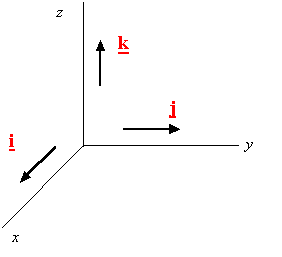Base vectors for a rectangular coordinate system: A set of three mutually orthogonal unit vectors

Right handed system: A coordinate system represented by base vectors which follow the right-hand rule.

Rectangular component of a Vector: The projections of vector A along the x, y, and z directions are Ax, Ay, and Az, respectively.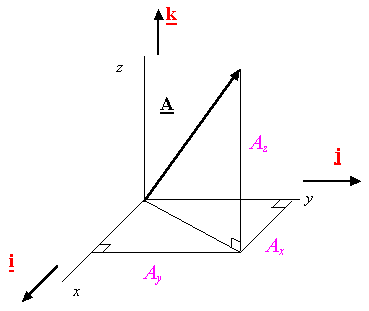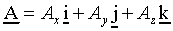Magnitude of a Vector: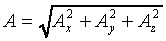Direction Cosines: Cos(a), Cos(b), Cos(g)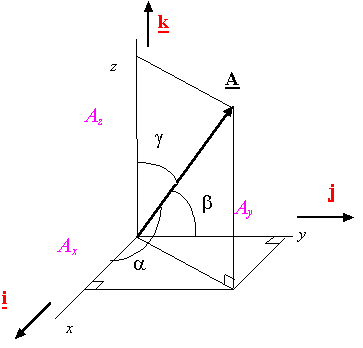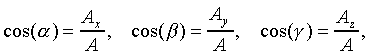Unit vector along a vector: The unit vector uA along the vector A is obtained from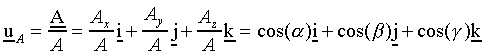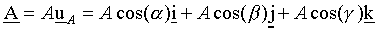Addition of vectors: The resultant vector FR obtained from the addition of vectors F1, F2, …, Fn is given by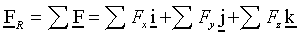Coordinates of points in space: The triplet (x,y,z) describes the coordinates of a point.

The vector connecting two points: The vector connecting point A to point B is given by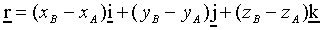A unit vector along the line A-B: A unit vector along the line A-B is obtained from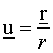A vector along A-B: A vector F along the line A-B and of magnitude F can be obtained from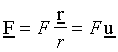The dot product: The dot product of vectors A and B is given by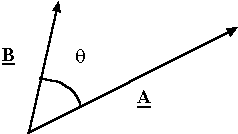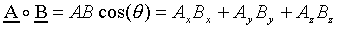Projection of a vector by using the dot product: The projection of vector A along the unit vector u is given by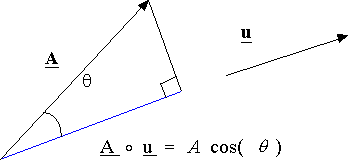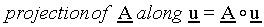Examples: